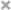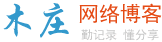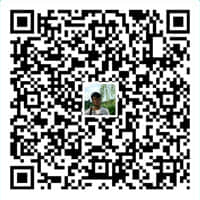# input输入框只能输入数字、字母相关组合（正则表达式）( 推荐学习：html教程 )

 1234567891011121314151617181920212223242526272829 ```<``html``>``    ``<``meta` `charset``=``"utf-8"` `/>``    ` `    ``<``body``>只允许输入正整数：``        ``<``input` `type``=``'text'` `onkeyup``=``"this.value=this.value.replace(/^(0+)|[^\d]+/g,'')"``>``        ``<``br``/>``        ``<``br``/>只允许输入英文:``        ``<``input` `type``=``"text"` `onkeyup``=``"this.value=this.value.replace(/[^a-zA-Z]/g,'')"``>``        ``<``br``/>``        ``<``br``/>只允许允许输入数字和字母：``        ``<``input` `onKeyUp``=``"value=value.replace(/[\W]/g,'')"``>``        ``<``br``/>``        ``<``br``/>允许输入大小写字母、数字、下划线：``        ``<``input` `type``=``"text"` `onkeyup``=``"this.value=this.value.replace(/[^\w_]/g,'');"``>``        ``<``br``/>``        ``<``br``/>允许输入小写字母、数字、下划线：``        ``<``input` `type``=``"text"` `onkeyup``=``"this.value=this.value.replace(/[^a-z0-9_]/g,'');"``>``        ``<``br``/>``        ``<``br``/>允许输入数字和小数点：``        ``<``input` `type``=``"text"` `onkeyup``=``"this.value=this.value.replace(/[^\d.]/g,'')"``>``        ``<``br``/>``        ``<``br``/>允许输入中文、数字、英文:``        ``<``input` `onkeyup``=``"value=value.replace(/[^\w\u4E00-\u9FA5]/g, '')"``>``        ``<``br``/>``        ``<``br``/>``    ``` ``

html中如何设置不可点击

html的 <nav> 标签

html怎么输入多行文字

html input只输入数字怎么设置

html pre标签怎么用

html无法播放视频怎么办

html实现在消息按钮上增加数量角标的实现代码

html中hr怎么设置粗细

html span标签怎么用### 评论

• 欢迎访问木庄网络博客
• 可复制：代码框内的文字。
• 方法：Ctrl+C。